# How to invert the elements of a boolean array in Python?

Sometimes, the task is to invert a boolean array. This is often required if the files contain a column with true or false values and the need is to use the column values in the inverted manner. For example, if a CSV file contains the data column as Covid Negative status showing True for covid –ve patients and the need is to create a column of covid+ve status. In such cases, the array inversion for the boolean values is required. In this Python article, using four different examples, the different ways to invert a Boolean array with or without using numpy, are given. Also, the plots representing boolean values from both the original array and the inverted one are shown in this article.

### The data.csv File used for Getting the Boolean Column data

Patientname,covidnegative
Raju,True
Meena,False
Happy,True
……,……


## Example 1: Using Numpy to Invert a Boolean Array and Compare the Boolean Value Plots

### Algorithm

• Step 1 − First import pandas, numpy and plotly. Plotly is the open source graphing library for Python that will be used for making scatter plots.

• Step 2 − Now read the data.csv file, convert it to dataframe, and make a scatter plot showing the boolen column “covidnegative” on the Y axis.

• Step 3 − Read the Boolean column values. Now using numpy functions convert it to an array and then invert the array using numpy’s invert function.

• Step 4 − Add a new column “covidpositive” into the dataframe and add the inverted values.

• Step 5 − Make a scatter plot again, showing the boolean column “covidpositive” on the Y axis.

• Step 6 − Write the function to show the scatterplot. Run the program using the cmd window. The plots will open in a new tab in the browser. Compare the y-axis of both plots.

#include the required libraries
import pandas as pd
import numpy as np

#This library is needed to make the scatter plot
import plotly.express as pxx

#read the csv file and make a dataframe

#print the colums and data
#make the scatter plot
figg = pxx.scatter(dff, x="patientname", y="covidnegative")

#set the properties of the scatter plot
figg.update_traces(marker=dict(size=12, line=dict(width=2, color="blue")), selector=dict(mode='markers'))

#display the chart
figg.show()
covidstatus=dff["covidnegative"]
arr = covidstatus.to_numpy()

#print(arr)
arr_inver = np.invert(arr)

#print(arr_inver)
dff['covidpositive'] = arr_inver.tolist()

#print(dff)
#make the scatter plot
figg = pxx.scatter(dff, x="patientname", y="covidpositive")

#set the properties of the scatter plot
figg.update_traces(marker=dict(size=12, line=dict(width=2, color="red")), selector=dict(mode='markers'))

#display the chart
figg.show()


## Output

Run the Python file in the Command window −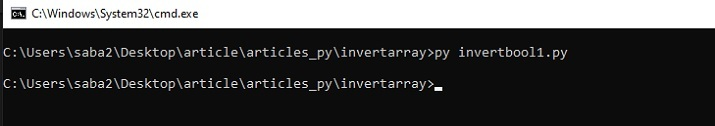The following figure shows the plots for the booean values in the original and inverted array.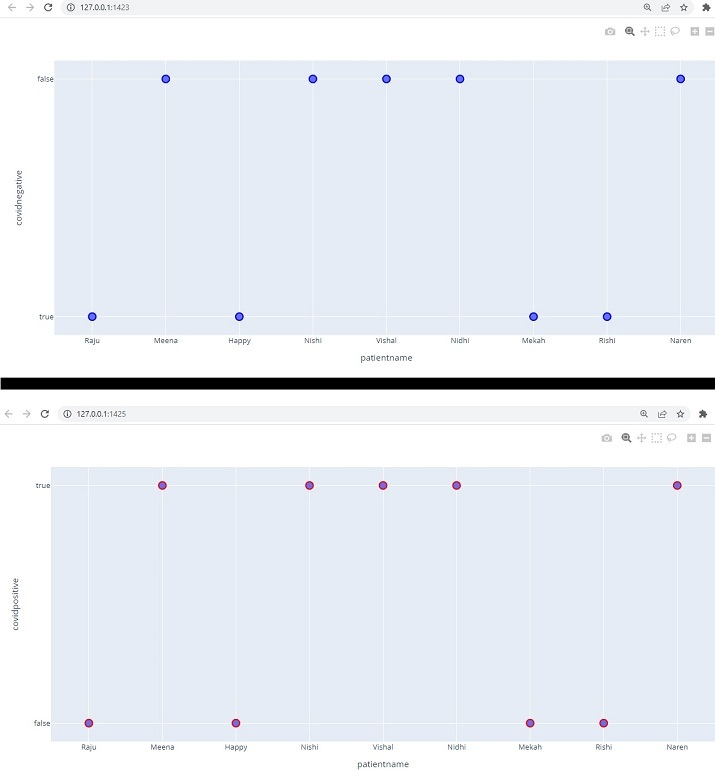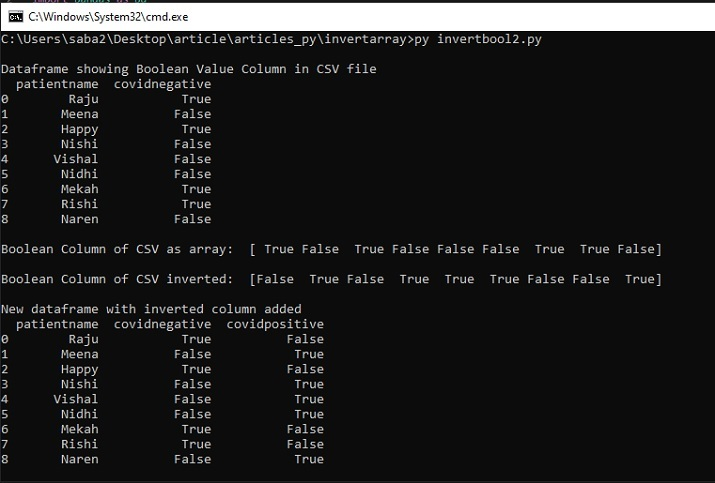## Example 2: Using  ~ on Numpy’s Array for Inverting Boolean Value Array

### Algorithm

• Step 1 − First import pandas and numpy.

• Step 2 − Now read the data.csv file, convert it to a dataframe, and print the data frame.

• Step 3 − Read the Boolean column values. Now using numpy functions convert it to an array and then invert the array using ~ on numpy’s array.

• Step 4 − Add a new column “covidpositive” into the dataframe and add the inverted values. Print the dataframe again.

• Step 5 − Run the program using cmd window. Compare the original and the inverted value Boolean columns.

#include the required libraries
import pandas as pd
import numpy as npp

#read the csv file and make a dataframe
print("\nDataframe showing Boolean Value Column in CSV file")
print(dff)
covidstatus=dff["covidnegative"]
arr01 = covidstatus.to_numpy()
print("\nBoolean Column of CSV as array: ")
print(arr01)
inverted_arr01 = ~npp.array(arr01, dtype=bool)
print("\nBoolean Column of CSV as inverted array : ")
print(inverted_arr01)
dff['covidpositive'] = inverted_arr01.tolist()
print("\nNew dataframe with inverted column added :")
print(dff)


## Output

Run the Python file in the Command window −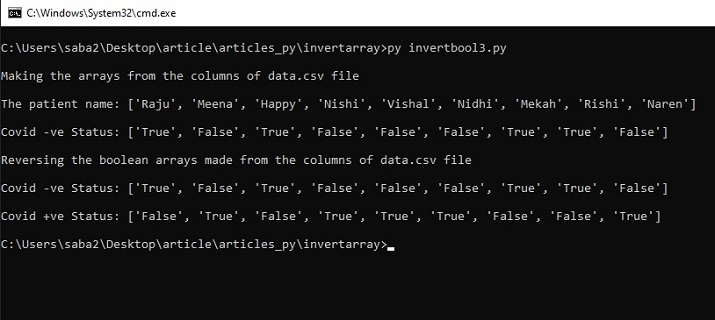## Example 3: Using  Numpy’s Logical_not for Inverting Boolean Value Array

### Algorithm

• Step 1 − First import pandas and numpy.

• Step 2 − Now read the data.csv file, and convert it to dataframe.

• Step 3 − Read the Boolean column values. Now using numpy functions convert it to an array and print it. Now invert the array using logical_not on NumPy’s array and print it again.

• Step 4 − Add a new column “covidpositive” into the dataframe and add the inverted values.

• Step 5 − Run the program using the cmd window. Compare the original and the inverted value in both arrays.

#include the required libraries
import pandas as pd
import numpy as npp

#read the csv file and make a dataframe
covidstatus=dff["covidnegative"]
arr01 = covidstatus.to_numpy()
print("\nA given boolean array: ", arr01)
arr_inver = npp.logical_not(arr01)
print("\nThe inverted boolean array: ", arr_inver)
dff['covidpositive'] = arr_inver.tolist()

#print(dff)


## Output

Run the Python file in the Command window −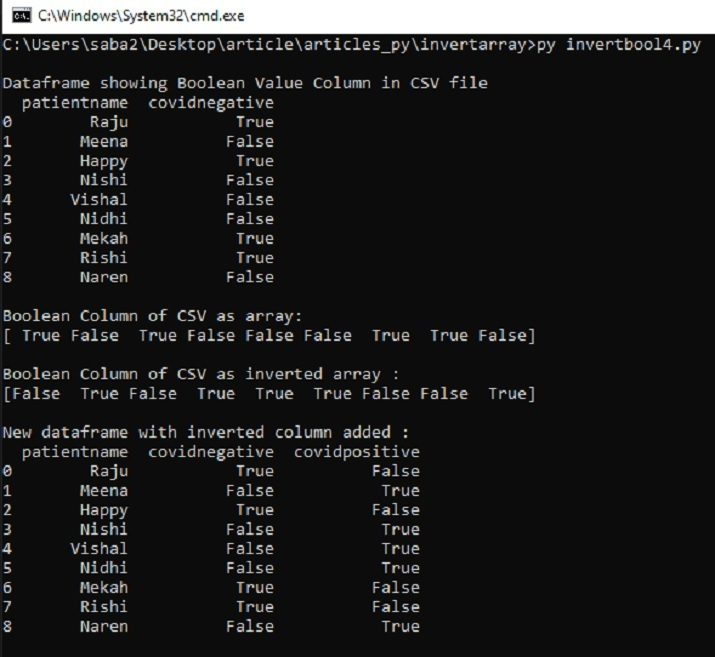## Example 4: Without Using  Numpy Inverting a Boolean Value Array

### Algorithm

• Step 1 − Import CSV and then use csv.DictReader to read the CSV file with Boolean value column.

• Step 2 − Make separate empty arrays to hold the values from columns of the CSV file and append the column values to these arrays.

• Step 3 − Define a function “reverse_bool” to convert true to false and false to true and return the inverted values.

• Step 4 − Using the map function use the “reverse_bool” function to convert each false to true and true to false.

• Step 5 − Run the program using the cmd window. Compare the original and the inverted value in both arrays.

invertbool3.py
# importing the module
import csv

# open the file in read mode
filename01 = open('data.csv', 'r')

# creating empty lists
covidstatus = []
name=[]
def reverse_bool(status):
if status == "True":
return "False"
elif status == "False":
return "True"
print('\nMaking the arrays from the columns of data.csv file')
# iterating over each row and append
# values to empty list
for col in file01:
covidstatus.append(col['covidnegative'])
name.append(col['patientname'])
# printing lists
print('\nThe patient name:',name)
print('\nCovid -ve Status:', covidstatus)
print('\nReversing the boolean arrays made from the columns of data.csv file')
resultbool = list(map(reverse_bool, covidstatus))
print('\nCovid -ve Status:', covidstatus)
print('\nCovid +ve Status:', resultbool)


## Output

Run the python file in the Command window −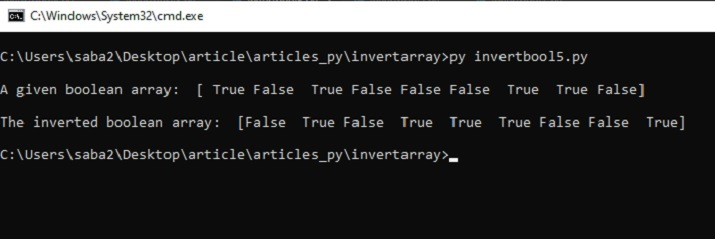In this Python article, by four different examples, the ways to show how to invert a Boolean array are given. The first three methods use NumPy’s functions for inverting the Boolean values. Then a Python program is written to make the inverted array without using numpy. In the first example, the scatter plots are also compared for showing the Boolean original values as well as the inverted values.

Updated on: 04-May-2023

243 Views